×

Search anything:

# Relational Operators for comparing Queue in C++ STL

#### Software Engineering C++Get this book -> Problems on Array: For Interviews and Competitive Programming

Reading time: 30 minutes | Coding time: 10 minutes

In this article, we will take a look at different relational operators for Queue container in C++ STL. This enables a programmer to compare queue directly. Some of the relational operators are:

• `==`
• `!=`
• `<`
• `<=`
• `>`
• `>=`

Queue is a linear data structure that implements FIFO(First In First Out).Queue comes under Sequence container adpaters of C++ container library.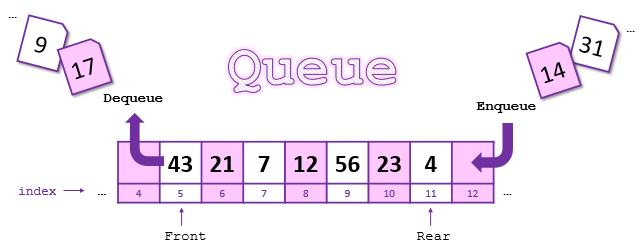Relational Operators used with queue c++ STL are overloaded operators which are used to perform appropriate comparisons between two queue objects. The queues are compared element by element.

Relational Operators for Queue are -

## (1) ==

``````bool operator== (const queue<T,Container>& queue1,const queue<T,Container>& queue2);
``````

It returns true if queue 1 is identical to queue 2, otherwise false.

The queue gets compared element by element. First the element 1 queue 1 gets compared to element 1 of queue 2 , Then element 2 of queue 1 gets compared to element 2 of queue 2 and so on. If all the elements are found to be same and length of both queues is equal then true is returned , Otherwise false.

### Example 1.1

``````#include <iostream>
#include <queue>

using namespace std;
int main()
{
queue<int> q1, q2;
q1.push(1); //pushing 1 into q1  ,Now q1= 1
q2.push(1); //pushing 1 into q2  ,Now q2= 1

if (q1 == q2)/*q1 and q2 contains same elements and queues are of same length. Hence q1 == q2 returns true*/
cout << "\n q1 and q2 are same";
else
cout<<"\nq1 and q2 are different";
return 0;
}
``````

Output: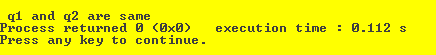### Example 1.2

``````#include <iostream>
#include <queue>

using namespace std;
int main()
{
queue<int> q1, q2;
q1.push(1); //pushing 1 into q1  ,Now q1= 1
q2.push(2); //pushing 1 into q2  ,Now q2= 1

if (q1 == q2)//q1 and q2 contains different elements.Hence q1 == q2 returns false
cout << "\n q1 and q2 are same";
else
cout<<"\nq1 and q2 are different";
return 0;
}
``````

Output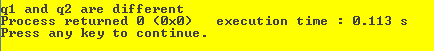## (2) !=

``````bool operator!= (const queue<T,Container>& queue1,const queue<T,Container>& queue2);
``````

It returns true if queue 1 is not identical to queue 2, otherwise false.

The queue gets compared element by element. First the element 1 queue 1 gets compared to element 1 of queue 2 , Then element 2 of queue 1 gets compared to element 2 of queue 2 and so on. If any element is found to be not equal then true is returned , Otherwise false.

### Example 2.1

``````#include <iostream>
#include <queue>

using namespace std;
int main()
{
queue<int> q1, q2;
q1.push(1); //pushing 1 into q1  ,Now q1= 1
q2.push(1); //pushing 1 into q2  ,Now q2= 1
q2.push(2); //pushing 2 into q2  ,Now q2= 1 2

if (q1 != q2)//q1 and q2 contains different elements.Hence q1 != q2 returns true
cout << "\n q1 and q2 are not same";
else
cout<<"\nq1 and q2 are same";
return 0;
}
``````

Output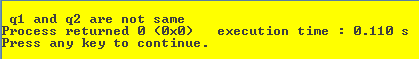### Example 2.2

``````#include <iostream>
#include <queue>

using namespace std;
int main()
{
queue<int> q1, q2;
q1.push(1); //pushing 1 into q1  ,Now q1= 1
q2.push(1); //pushing 1 into q2  ,Now q2= 1
q2.push(2); //pushing 2 into q2  ,Now q2= 1 2
q1.push(2); //pushing 2 into q1  ,Now q1= 1 2

if (q1 != q2)//q1 and q2 contains same elements.Hence q1 != q2 returns false
cout << "\n q1 and q2 are not same";
else
cout<<"\nq1 and q2 are same";
return 0;
}
``````

Output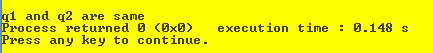## (3) <

``````bool operator<  (const queue<T,Container>& queue1,const queue<T,Container>& queue2);
``````

It returns true if queue 1 is less than queue 2, otherwise false.

The queue gets compared element by element. First the element 1 queue 1 gets compared to element 1 of queue 2 , Then element 2 of queue 1 gets compared to element 2 of queue 2 and so on. If all the elements of queue 1 are found to be less than elements of queue 2 then true is returned , Otherwise false.

### Example 3

``````#include <iostream>
#include <queue>

using namespace std;
int main()
{
queue<int> q1, q2;
q1.push(1); //pushing 1 into q1  ,Now q1= 1
q2.push(1); //pushing 1 into q2  ,Now q2= 1
q1.push(2); //pushing 2 into q2  ,Now q1= 1 2
q2.push(3); //pushing 3 into q1  ,Now q2= 1 3

if (q1 < q2)//q2 has element greater than q1.Hence q1 < q2 returns true
cout << "\n q1 is less than q2";
else
cout<<"\nq1 is greater than q2";
return 0;
}
``````

Output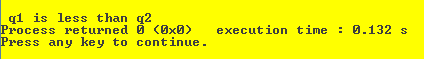## (4) <=

``````bool operator<= (const queue<T,Container>& Queue1,const queue<T,Container>& Queue2);
``````

It returns true if queue 1 is less than or equal to queue 2, otherwise false.

The queue gets compared element by element. First the element 1 queue 1 gets compared to element 1 of queue 2 , Then element 2 of queue 1 gets compared to element 2 of queue 2 and so on. If all the elements of queue 1 are found to be less than or equal to elements of queue 2 then true is returned , Otherwise false.

### Example 4

``````#include <iostream>
#include <queue>

using namespace std;
int main()
{
queue<int> q1, q2;
q1.push(1); //pushing 1 into q1  ,Now q1= 1
q2.push(1); //pushing 1 into q2  ,Now q2= 1
q1.push(2); //pushing 2 into q2  ,Now q1= 1 2
q2.push(3); //pushing 3 into q1  ,Now q2= 1 3

if (q1 <= q2)//q2 has element greater than q1.Hence q1 < q2 returns true
cout << "\n q1 is less than equal to q2";
else
cout<<"\nq1 is greater than q2";
return 0;
}
``````

Output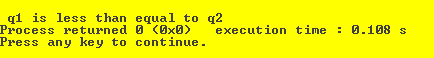## (5) >

``````bool operator>  (const queue<T,Container>& Queue1,const queue<T,Container>& Queue2);
``````

It returns true if queue 1 is greater than queue 2, otherwise false.

The queue gets compared element by element. First the element 1 queue 1 gets compared to element 1 of queue 2 , Then element 2 of queue 1 gets compared to element 2 of queue 2 and so on. If all the elements of queue 1 are found to be greater than elements of queue 2 then true is returned , Otherwise false.

### Example 5

``````#include <iostream>
#include <queue>

using namespace std;
int main()
{
queue<int> q1, q2;
q1.push(1); //pushing 1 into q1  ,Now q1= 1
q2.push(1); //pushing 1 into q2  ,Now q2= 1
q1.push(5); //pushing 2 into q2  ,Now q1= 1 5
q2.push(4); //pushing 3 into q1  ,Now q2= 1 4

if (q1 > q2)//q1 has element greater than q2.Hence q1 > q2 returns true
cout << "\n q1 is greater than q2";
else
cout<<"\nq1 is less than q2";
return 0;
}
``````

Output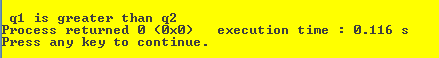## (6) >=

``````bool operator>= (const queue<T,Container>& Queue1,const queue<T,Container>& Queue2);
``````

It returns true if queue 1 is greater than or equal to queue 1, otherwise false.

The queue gets compared element by element. First the element 1 queue 1 gets compared to element 1 of queue 2 , Then element 2 of queue 1 gets compared to element 2 of queue 2 and so on. If all the elements of queue 1 are found to be greater than or equal to elements of queue 2 then true is returned , Otherwise false.

### Example 4

``````#include <iostream>
#include <queue>

using namespace std;
int main()
{
queue<int> q1, q2;
q1.push(1); //pushing 1 into q1  ,Now q1= 1
q2.push(1); //pushing 1 into q2  ,Now q2= 1
q1.push(2); //pushing 2 into q2  ,Now q1= 1 2
q2.push(3); //pushing 3 into q1  ,Now q2= 1 3

if (q1 <= q2)//q2 has element greater than q1.Hence q1 < q2 returns true
cout << "\n q1 is less than equal to q2";
else
cout<<"\nq1 is greater than q2";
return 0;
}
``````

Output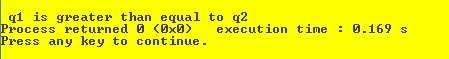All above relational operators return True if the condition holds, and False otherwise.

The time complexity of all above relational operators are O(N).

### Working

The two queues are compared by comparing their values pairwise, starting at the beginning, with each comparison looking at two values in corresponding positions, until a determination of the appropriate relationship between the two queues can be made.

### Code Example

``````#include <iostream>
#include <queue>

using namespace std;

int main()
{
queue<int> q1, q2;  //creating two queue objects
cout<<"Relational Operators for Queue";

for(int i=1;i<=5;i++)
{
q1.push(i);    //pushing values (1-5) into queue 1
q2.push(i);    //pushing values (1-5) into queue 2
}

if (q1 == q2)  //returns true as both the queues contain same elements
cout << "\nq1 and q2 are same";

q1.push(6);     //pushing value 6 into queue 1
q2.push(20);

/*Now queue 1 is 1 2 3 4 5 6 and queue 2 is 1 2 3 4 5 20
making queue 1 less than queue 2*/

if (q1 != q2)
cout << "\nq1 and q2 are not same";
if(q1 < q2)
cout<<"\nq1 is less than q2";
if(q1 <= q2)
cout<<"\nq1 is less than equal to q2";
if(q1 > q2)
cout<<"\nq1 is greater than q2";
if(q1 >= q2)
cout<<"\nq1 is greater than equal to q2";

return 0;
}
``````

Output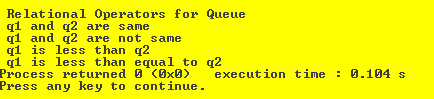### Applications

• Relational Operators for queue helps us compare elements of queue.
• It makes it easier for us programmers to do so as we don't have to write the same set of code to perform relational operations in every queue based projects.

## Question

Consider the following C++ code:

``````#include <iostream>
#include <queue>

using namespace std;

int main()
{
queue<int> q1, q2;
q1.push(0);
q2.push(NULL);
if (q1 == q2)
cout << "\n q1 and q2 are same";
return 0;
}
``````

#### What will be the output of the above code?

###### What is the output of above C++ program?
q1 and q2 are same
Compilation error as Null cannot be pushed
Compilation error as q1 and q2 cannot be compared
Run time error

#### Saatwik Bisaria

Bachelor of Technology in Information Technology at Bharati Vidyapeeth's College of Engineering